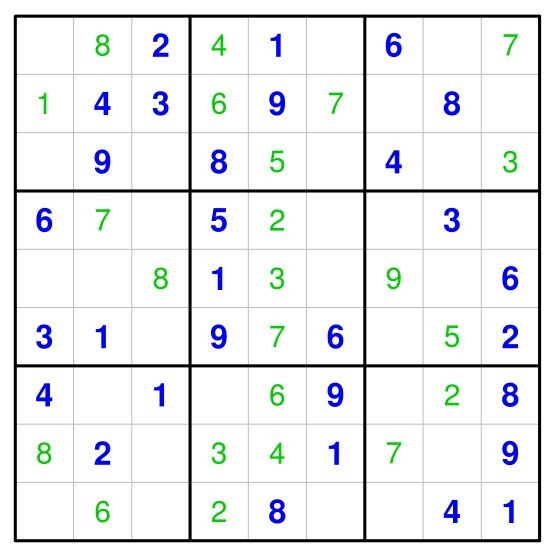# 一、简述交互式作图

## 1.1 键盘信息的获取

getGraphicsEvent(prompt = "Waiting for input",
onMouseDown = NULL, onMouseMove = NULL,
onMouseUp = NULL, onKeybd = NULL,
consolePrompt = prompt)


par(bty = "n", xaxt = "n", yaxt = "n", mar = c(0, 0, 0, 0), cex = 4,
font = 4)
plot(c(0, 1), c(0, 1), type = "n", xlab = "", ylab = "")
num <- 1:99
random1 <- function(key) {
if (key == "a") {
rect(0.2, 0.2, 0.8, 0.8, col = "white", border = NA)
B <- sample(num, 1)
text(0.5, 0.5, B, col = "blue", cex = 4)
}
}
getGraphicsEvent("run", onKeybd = random1)


## 1.2 鼠标信息的获取

### 1.2.1 位置信息

locator(n = 512, type = "n", ...)


par(bty = "n", xaxt = "n", yaxt = "n", mar = c(0, 0, 0, 0), cex = 5,
font = 4)
plot(c(0, 1), c(0, 1), type = "n", xlab = "", ylab = "")
locator(n = 5, type = "l", lwd = 2, col = sample(1:7, 1))


identify(x, y = NULL, labels = seq_along(x), pos = FALSE, n = length(x),
plot = TRUE, atpen = FALSE, offset = 0.5, tolerance = 0.25,
...)


par(bty = "n", xaxt = "n", yaxt = "n", mar = c(0, 0, 0, 0), font = 4)
plot(c(0, 1), c(0, 1), type = "n", xlab = "", ylab = "")
xx <- runif(100)
yy <- runif(100)
identify(xx, yy)


### 1.2.2 动作信息

par(bty = "n", xaxt = "n", yaxt = "n", mar = c(0, 0, 0, 0), cex = 5,
font = 4)
plot(c(0, 1), c(0, 1), type = "n", xlab = "", ylab = "")
move <- function(button, x, y) {
points(x, y, cex = 2, col = sample(1:7))
}
getGraphicsEvent("", onMouseMove = move)


# 二、游戏界面制作

## 2.1 par函数

par(bty = "n", xaxt = "n", yaxt = "n", mar = c(0, 0, 0, 0), cex = 5,
font = 4)


par是一个极其重要的设置图形参数(当然也可以获取)的函数，尤其是对于非常想追求作图效果的user而言(ggplt2脑残粉可暂时忽略这句话)。随便举个例子，总不可能在游戏界面中出现一个坐标系吧，那难免也太丧心病狂了，这也就是bty=“n”的意义之所在了。因此做界面的一个首要条件是要学会设置，也就是会运用par函数(很多设置在plot等绘图函数也能实现)，par函数参数众多，覆盖面很全，想详细了解的仔细阅读帮助文件即可。另外谢大在他未完成的在线著作《现代统计图形》中也有对par函数和plot函数详细解读，还包括介绍哪些参数是只能在par 中设置的，可以说是提取了帮助文件中的精华部分翻译后展现出来，很有学习价值。

## 2.2 低级绘图函数

plot(c(0, 1), c(0, 1), type = "n", xlab = "", ylab = "")


## 2.4 写function能事半功倍

plot.flag <- function(x, y, ...) {
polygon(c(x - 0.1, x - 0.1, x + 0.25, x + 0.25), c(y + 0.1,
y + 0.3, y + 0.3, y + 0.1), col = "red", border = "red")
polygon(c(x - 0.3, x - 0.3, x + 0.3, x + 0.3), c(y - 0.3,
y - 0.2, y - 0.2, y - 0.3), col = "black", border = "black")
segments(x - 0.1, y + 0.3, x - 0.1, y - 0.2)
}


# 三、示例-瞻仰COS前辈

## 3.1 (围棋)五子棋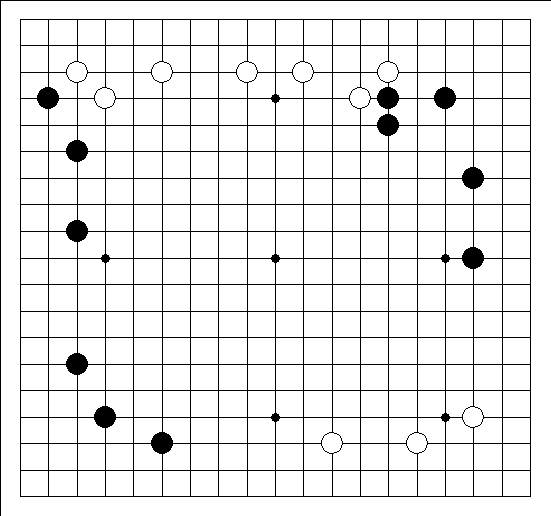## 3.2 扫雷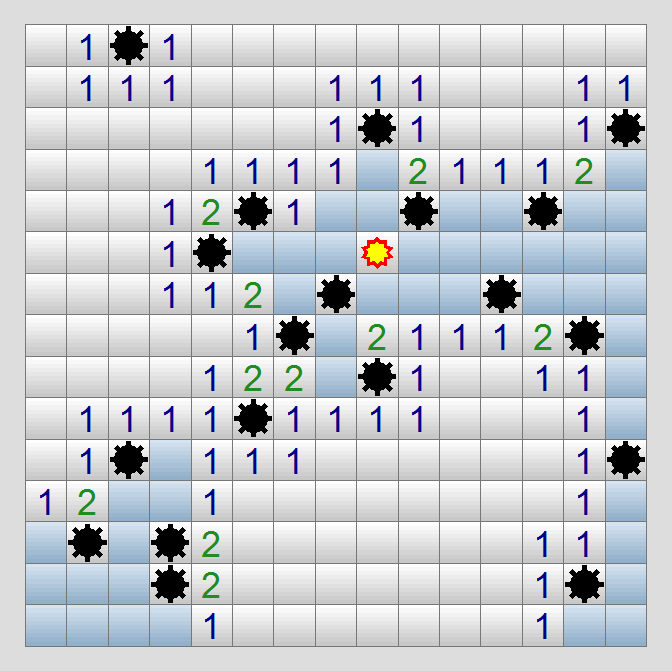## 3.3 关灯游戏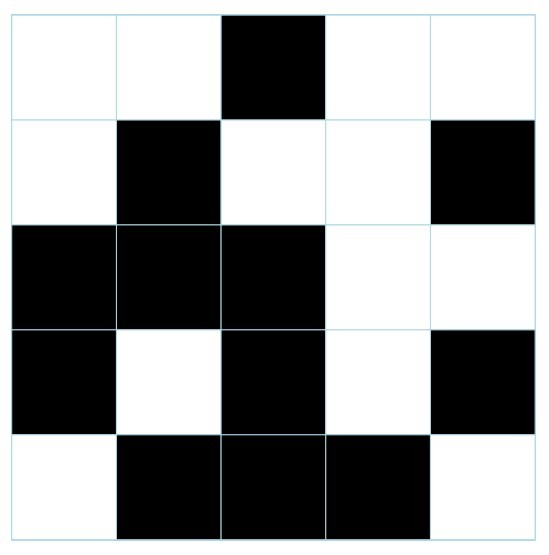# 四、示例——如何DIY？

## 4.1 抽奖装置

par(bty = "n", xaxt = "n", yaxt = "n", mar = c(0, 0, 0, 0), cex = 4,
font = 4)
plot(c(0, 1), c(0, 1), type = "n", xlab = "", ylab = "")
text(0.4, 0.9, "Who's turn?", col = rainbow(1000)[sample(1:1000,
1)], cex = 1)
text(0.8, 0.1, "made by Chen-ang Liu", col = rainbow(1000)[sample(1:1000,
1)], font = 2, cex = 0.2)
num <- 1:99
A <- 100
run <- function(key) {
if (key == "s") {
rect(0.2, 0.2, 0.8, 0.8, col = "white", border = NA)
B <- sample(num, 1)
A <- c(A, B)
text(0.5, 0.5, B, col = rainbow(1000)[sample(1:1000,
1)], cex = 4)
}
}
getGraphicsEvent("run", onKeybd = run)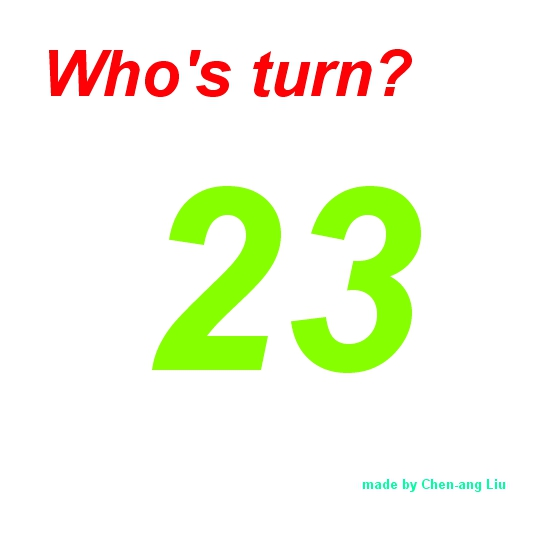# 五、一条龙之作——sudoku包

> playSudoku()
Solving...done!
? -- this help
1-9 -- insert digit
0,' ' -- clear cell
r -- replot the puzzle
q -- quit
h -- hint/help
c -- correct wrong entries (show in red)
u -- undo last entry
s -- show number in cell
a -- show all (solve the puzzle)

Ready!